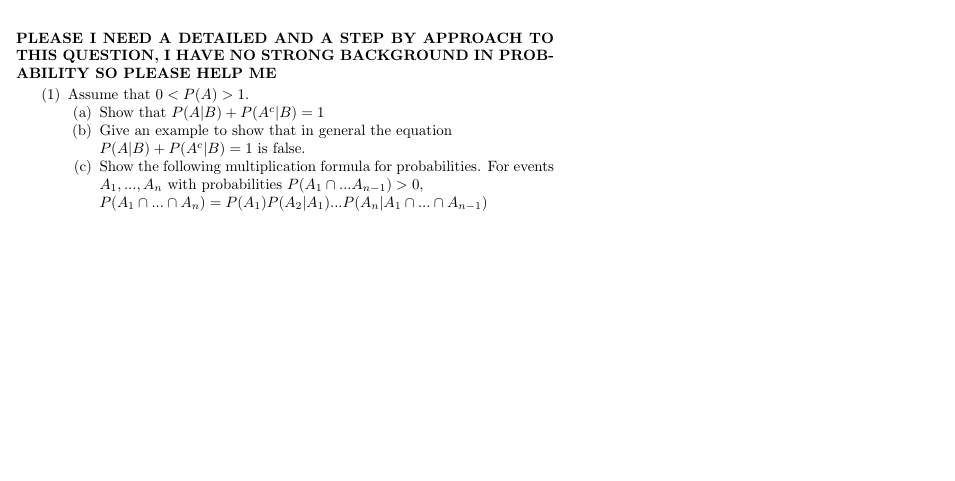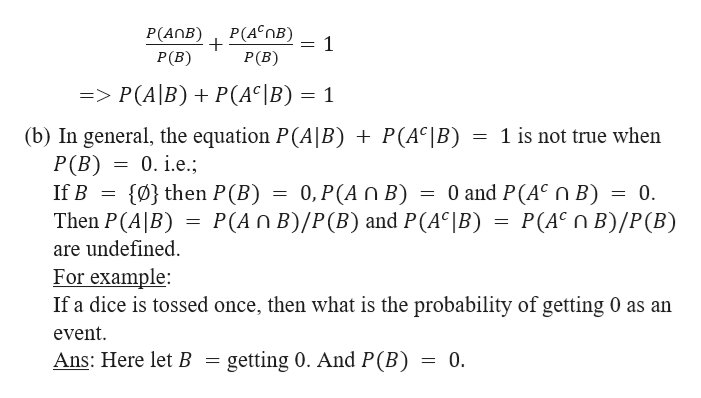PLEASE I NEED A DETAILED AND A STEP BY APPROACH TOTHIS QUESTION, 1 HAVE NO STRONG BACKGROUND IN PROB-ABILITY SO PLEASE HELP ME(1) Assume that 0 < P(A) 1.(a) Show that P(A|B) P(Ac|B) 1(b) Give an example to show that in general the equationP(A|B) P(AcB) 1 is false(c) Show the following multiplication formula for probabilities. For eventsA,. An with probabilities P(A1...An-1)> 0P(A nn An) = P(A)P(A2|A1)...P(A,|A1 n...n An-1)

Question

Please I need a step by step approach to these problem. Thank youhelp_outlineImage TranscriptionclosePLEASE I NEED A DETAILED AND A STEP BY APPROACH TO THIS QUESTION, 1 HAVE NO STRONG BACKGROUND IN PROB- ABILITY SO PLEASE HELP ME (1) Assume that 0 < P(A) 1. (a) Show that P(A|B) P(Ac|B) 1 (b) Give an example to show that in general the equation P(A|B) P(AcB) 1 is false (c) Show the following multiplication formula for probabilities. For events A,. An with probabilities P(A1...An-1)> 0 P(A nn An) = P(A)P(A2|A1)...P(A,|A1 n...n An-1) fullscreen
Step 1

(a) Let us assume that P(A), P(B) > 0.

We know P(A|B) = P(A∩B)/P(B).----------------(1)

We need to show that P(A|B) + P(Ac|B) = 1

From the fig below, it can be seen that,

B = (A∩B)∪( Ac∩B )

=> P(B) = P(A∩B) + P(Ac∩B)

=>P(A∩B)/P(B) + P(Ac∩B)/P(B) = 1

Step 2

So,         &n...help_outlineImage TranscriptioncloseP(AnB 4*nB) . P(B) P(B) 1 => P(A|B) P(AC|B) = 1 (b) In general, the equation P(A|B) + P(AC|B) = 1 is not true when P (B) 0.i.e. 0 and P(AC n B) = 0 = P(AC B)/P(B) (0} then P (B) If B 0, P(A n B) P(An B)/P(B) and P(AC|B) Then P(A|B) are undefined For example If a dice is tossed once, then what is the probability of getting 0 as an event Ans: Here let B getting 0. And P(B) = 0 fullscreen

Want to see the full answer?

See Solution

Want to see this answer and more?

Our solutions are written by experts, many with advanced degrees, and available 24/7

See Solution
Tagged in

Basic Probability